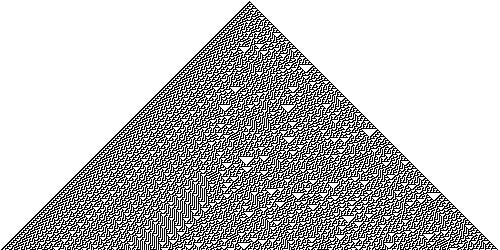## Introduction

Stephen Wolfram’s elementary cellular automata are something I read about a while ago and thought looked quite cool. Cellular automata, in general, are grids of cells which evolve over time without any external input. I decided to write a small Ruby program that would be capable of calculating a new generation for any elementary cellular automaton given an initial state.

This is the code I ended up with:

``````def evolve(rule, state)
def state.[](x)
(x < 0 or x >= length) ? 0 : fetch(x)
end
next_state = []
for i in (-1 ... state.length + 1)
bit = state[i + 1] | (state[i] << 1) | (state[i - 1] << 2)
next_state[i + 1] = ((mask & rule) != 0) ? 1 : 0
end
return next_state
end
``````

I think it illustrates some of the nice features from Ruby, such as metaprogramming and the clean syntax so I decided to put it up here with a bit of an explanation.

``````def evolve(rule, state)
def state.[](x)
(x < 0 or x >= length) ? 0 : fetch(x)
end
``````

The first line defines the method, `evolve()`, which is passed the rule number and an array containing the current state. The array should simply contain a sequence of 0s and 1s.

The next three lines add a method only to the `state` object. This method overrides the array access operator, so that indices outside of the array always return 0, as opposed to the normal Ruby behaviour. This simplifies access to the array later on.

``````  next_state = []
for i in (-1 ... state.length + 1)
``````

The next line simply creates a new array for the new state. Then, all of the indices inside the current state array as well as one before it and after it are looped through by the for loop. This is done because for each generation the array of cells becomes larger by one cell at each end.

``````    bit = state[i + 1] | (state[i] << 1) | (state[i - 1] << 2)
``````

This snippet takes the values of the cells to the top left, top middle and top right above the current cell and turns it into a single number using bit shifts. This number can be used to find the next state of the cells.

For example, in Rule 30, if the current state is 100 in binary, this is the 4th bit. 30 in binary is 00011110, so the next state would be 1.

``````    mask = 1 << bit
next_state[i + 1] = ((mask & rule) != 0) ? 1 : 0
``````

This code actually implements the above check using the AND operator. If we want to test the 5th bit, we perform 2^4 (equivalent to 1 « 4) which gives 16. By performing 30 AND 16 we get a non-zero value, which indicates the next state is indeed 1.

``````  end
return next_state
end
``````

The last few lines simply terminate the loop, return the state and end the method.

## ASCII art

I then wrote a quick program to test this by drawing ASCII art:

``````rule = ARGV.to_i
state = 
max = 64
max.times do |gen|
(max - gen).times { print ' ' }
state.each { |x| print x == 1 ? '*' : ' ' }
puts

state = evolve(rule, state)
end
``````

This is the first few lines of output it produces for rule 90, one of my favourites:

``````               *
* *
*   *
* * * *
*       *
* *     * *
*   *   *   *
* * * * * * * *
*               *
* *             * *
*   *           *   *
* * * *         * * * *
*       *       *       *
* *     * *     * *     * *
*   *   *   *   *   *   *   *
* * * * * * * * * * * * * * * *
``````

I really find it amazing that from a simple rule you can get such an elegant pattern (or with a different rule, you can get something that is chaotic or even turing-complete).

## Nature

What I find most startling about elementary cellular automata is that patterns built up from these simple rules can also be found in nature. For example, here is a picture of rule 30 and a species of sea snail that has a similar pattern on its shell:(photograph from Richard Ling, licensed under CC BY-SA 3.0)

## Conclusion

This post has probably seemed a bit rambling and pointless, but it was on my list of interesting things to talk about and if you haven’t seen these before then hopefully you’ll get the ‘oh, that’s quite cool’ moment too!